# 9.4 Relations between Cartesian, Cylindrical, and Spherical Coordinates

Consider a cartesian, a cylindrical, and a spherical coordinate system, related as shown in Figure 1.Figure 1: Standard relations between cartesian, cylindrical, and spherical coordinate systems. The origin is the same for all three. The positive z-axes of the cartesian and cylindrical systems coincide with the positive polar axis of the spherical system. The initial rays of the cylindrical and spherical systems coincide with the positive x-axis of the cartesian system, and the rays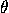=90° coincide with the positive y-axis.

Then the cartesian coordinates (x,y,z), the cylindrical coordinates (r,,z), and the spherical coordinates (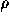,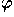,) of a point are related as follows: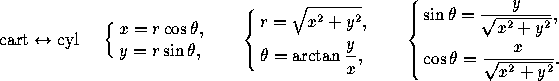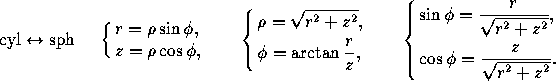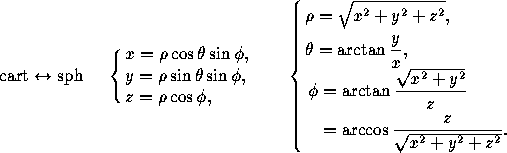Next: 9.5 Homogeneous Coordinates in Space
Up: 9 Coordinate Systems in Space
Previous: 9.3 Spherical Coordinates in SpaceThe Geometry Center Home Page

Silvio Levy
Wed Oct 4 16:41:25 PDT 1995

This document is excerpted from the 30th Edition of the CRC Standard Mathematical Tables and Formulas (CRC Press). Unauthorized duplication is forbidden.